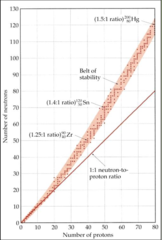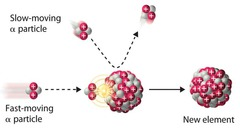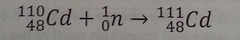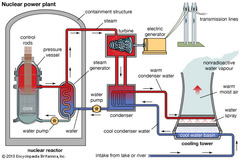# Chapter 20: Nuclear Chemistry EssayMary Moore
question

Nuclear chemistry

chemistry of the nucleus rather than the electrons.
question

Chemical Reaction

∙ does NOT change the nucleus ∙ different ISOTOPES behave essentially the SAME ∙rate is affected by temp, pressure, or catalyts ∙atoms in compounds behave differently than elements ∙relatively small energy change compared to nuclear reactions
question

Nuclear Reaction

∙ CHANGES the nucleus ∙ different ISOTOPES often behave DIFFERENTLY ∙rate is UNAFFECTED by temp, pressure (within the range found on Earth), or catalyst ∙atom behaves the SAME whether it is elemental or in a compound ∙energy change can be millions of times greater than a chemical reaction
question

nucleons

the nucleus is comprised of the two of these: protons and neutrons
question

atomic number

number of protons, determines element
question

mass number

number of protons and neutrons added together
question

isotopes

∙NOT all atoms of the same element have the same mass. ∙They can have DIFFERENT numbers of NEUTRONS, and thus different MASS numbers. ∙Atoms that have the same atomic number but different mass numbers are isotopes of each other
question

Spontaneous emission of particles or electromagnetic radiation from the nucleus The process is called *radioactive decay* or *nuclear decay.*
question

nuclear transmutation

bombardment of nuclei by neutrons, protons, or other nuclei.
question

Nuclei decay into different nucleus:

∙Alpha (a) decay: –>the nucleus loses TWO protons and TWO neutrons (i.e. helium nucleus) ∙Beta (b) decay: –>neutron→ proton + ejected electron ∙Gamma (g) emission: –>NO change in mass or atomic number ∙Positron emission (PE): –>proton→ neutron + ejected positron ∙electron capture (EC) –> proton + inner shell electron → neutron
question

The symbols for subatomic particles:

∙ proton ¹₁H ¹₁p ∙neutron ¹₀n ∙electron ⁰₋₁e ⁰₋₁B ∙positron ⁰⁺₁e ⁰⁺₁B ∙alpha particle ⁴₂a ⁴₂He
question

nuclear reactions

A nuclear equation is balanced when the number of nucleons and the sums of the charges are the same on both sides protons + neutrons→ protons→ 92 protons+ 146 neutrons 90 protons+ 144 neutrons 2 protons+ 2 neutrons
question

Nuclear Stability

**neutron-to-proton ratio (n/p) –> There are more stable nuclei with 2, 8, 20, 50, 82, or 126 protons or neutrons –>More with even #’s –>All with atomic number > 83 are radioactive –>All isotopes of ₄₃Tc and ₆₁Pm are radioactive
question

belt of stability∙Stable nuclei are located in this area of the graph ∙Most radioactive nuclei lie outside the belt. ∙Above the belt of stability, the nuclei have higher neutron-to-proton ratio.
question

Alpha Decay

∙the loss of an a-particle (a helium nucleus). ⁴₂He or ⁴₂a *The nucleus loses two protons and two neutrons
question

Beta decay

∙the loss of a B-particle (a high energy electron). no nucleons ↓ ⁰₋₁B or ⁰₋₁e
question

Beta emission

*decomposition of a neutron to yield an ELECTRON and a PROTON. –>Because it is from decomposition of a **neutron**, the ELECTRON is coming from the *nucleus*, NOT from the orbitals. –>It is ejected from the nucleus. –>atomic number INCREASES by 1 ₀¹n → ¹₁p + ⁰₋₁e (n→ p +) *e⁻*)→→→
question

∙the loss of a y-ray, which is made up of high-energy photons (electromagnetic radiation of very short wavelength). ∙Almost always accompanies the LOSS of a NUCLEAR particle, but is usually omitted from nuclear equations. ∙Emission of gamma rays causes NO change in mass or atomic number
question

Positron Emission

∙involves the conversion of a proton in the nucleus into a neutron plus an ejected positron. proton → neutron + positron ∙atomic number DECREASES by 1 ¹₁p → ¹₀n + ⁰₁e (p→ n + (*E+*)→→
question

positron

∙particle that has the same mass as but an opposite charge to that of an electron. ∙are very short-lived. When they collide with electrons, they are converted to gamma rays. positron + electron → 2 gamma rays →no nucleons⁰₁B or ⁰₁e
question

electron capture

∙electron is captured from the inner shell of the surrounding electron cloud. (¹₁p) proton + (⁰₋₁) electron → (¹₀n) neutron *atomic number DECREASES by 1
question

Nuclear Stability

∙Nuclei ABOVE the “belt of stability” have too many NEUTRONS. ∙They tend to decay by emitting *BETA particles.* ¹₀n → ¹₁p + ⁰₋₁e _______________________________ ∙Nuclei BELOW the belt have too many protons ∙They tend to become MORE STABLE by *positron emission* or *electron capture.* ¹₁p → ¹₀n + ⁰₁e or ¹₁p + ⁰₋₁e → ¹₀n ∙There are NO stable nuclei with an atomic number greater than 83 (no more “belt of stability”). i.e. All nuclei with more than 83 protons are radioactive. ∙Nuclei with such large atomic numbers (>83) tend to decay by *alpha emission.* ⁴₂He or ⁴₂a₂ ∙ Large nuclei emit large particles.
question

is a sequence of nuclear reactions that ultimately result in the formation of a stable isotope.
question

parent isotope

question

daughter isotope

the product isotope
question

Einstein’s famous equation expresses this:

E = mc² where E = energy, m = mass, and c = the speed of light (2.9979 x 10⁸ m/s). **The law of conservation of energy = The law of conservation of mass
question

nuclear binding energy

is the energy required to break up a nucleus into its component *protons and neutrons.*
question

nucleons (protons and neutrons)

The mass of a nucleus is always LESS than the masses of the individual nucleons (protons and neutrons) it is composed of. =this is called=> *mass defect*
question

Loss in mass is converted to energy and can be quantified with Einstein’s mass-energy equivalence relationship:

ΔE = (Δm)c² →ΔE = energy of product – energy of reactant →Δm = mass of product – mass of reactant ∙(= mass of nucleus – sum of mass of nucleons) ∙(= mass lost when nucleus is formed)
question

Nuclear Binding Energy

= -2.3686 x 10-11 J = energy lost (stability gained) when the nucleus of one atom is formed *the energy released when nucleons combine to form a nucleus; formation of the nucleus is energetically favorable *nuclear binding energy: the energy required to break up a nucleus into its component protons & neutrons
question

Binding Energy per Nucleon

(Nuclear Binding Energy) / (number of nucleons) **highest BEPN is the most stable (requires the most energy per nucleon to breakup the nucleus) **nuclei w/ mass number ~40-100 have the highest Binding Energy per Nucleon, and thus are the most stable.
question

∙Radioactive decay is a first-order process. ∙ The rate depends only on the number of radioactive nuclei N in the sample. Rate = kN where k is now called the *decay constant*,N is the number of *radioactive nuclei* in the sample
question

activity

∙rate at which the sample decays –>expressed in units of *bequerels (Bq)*, which equals 1 nuclear disintegration per second. –>*curie (Ci) * is 3.7 x 1010 disintegrations/second.
question

Integrated rate law:

ln Nt/N₀ = -kt where t= time interval of decay N₀= initial number of nuclei (at t=0) Nt= remaining number of nuclei after time t. –>Since mass and activity are each proportional to N, a *mass/mass ratio* or an *activity/activity ratio* can be used instead of N/N.
question

half-life, t½

is the time for half of the material to decay. So for t½ , Nt / N₀ = ½ and thus: ** 0.693/k = t₁/₂
question

Because the half-life of a particular nuclide is constant, by: *comparing the % abundance of a radioactive isotope at a given point in time with the % abundance normally present,* one can find the age of an object. ∙Living systems take up ¹⁴C, but no longer do when they die. ∙By measuring the amount of ¹⁴C present compared to the natural abundance of ¹⁴C, an approximate age of a fossil can be determined.
question

Nuclear transmutationsnuclear reactions induced by colliding a neutron or another nucleus with the nuclide. ²⁷₁₃Al + ¹₀n→ ²⁴₁₁Na + ⁴₂He
question

Shorthand way of summarizing a nuclear transmutation:

∙target nucleus (bombarding particle, ejected particle) product nucleus ²⁷₁₃Al (n,a) ²⁴₁₁Na ∙n is used for neutron, ∙p is used for proton, ∙a is used for alpha particle
question

Particle accelerators

Charged particles must be moving at high speed to have enough energy to *overcome charge-charge repulsion* and *collide with the nucleus.* –> A particle accelerator is used.
question

neutron captureCombination of a nucleus with a neutron takes place through this.
question

transuranium elements

∙Elements above atomic number 92 (Uranium) ∙ because they follow Uranium on the periodic table. All of them are radioactive ∙can be made by bombardment of smaller nuclei with neutrons or other nuclei. e.g. bombardment with an accelerated a-particle (a charged particle of 2 protons and 2 neutrons):
question

Nuclear Fission and Fusion

∙When small nuclei are *fused* into a larger nuclei of the same number of nucleons, *energy is released.* ∙ when large nuclei are fragmented *(fissioned)* into mid-sized nuclei with the same total number of nucleons, *energy is released.*
question

Nuclear fission

∙a heavy nucleus (mass number > 200) divides to form smaller nuclei and one or more neutrons. ∙Heavy nuclei gain stability (release energy) when fragmented into mid-sized nuclei. –> Bombardment of the radioactive nuclide with a neutron starts the process.
question

nuclear chain reaction

self-sustaining sequence of nuclear fission →Neutrons released in the transmutation strike other nuclei, causing their decay and the production of more neutrons.
question

critical mass

minimum amount of fissionable material present for the chain reaction to be sustained
question

Nuclear ReactorsIn nuclear reactors the heat generated by the reaction is used to produce steam that turns a turbine connected to a generator
question

control rods

capture some neutrons, keeping the system from reaching a dangerous supercritical mass
question

Light reactors

use water as a coolant and uranium-235 as fuel, usually in the form of U₃O₈. The U-235 must be enriched to 3-4%.
question

Heavy reactors

use D₂O, and the U-235 does not need to be enriched.
question

Breeder reactors

add U-238, plutonium-239, or thorium-232 to the U-235. Each of these produces more fissionable material as it absorbs neutrons.
question

Nuclear Fusion

Light nuclei are combined (fused) together to give heavier nuclei. –>Thermonuclear reactions: must be in the plasma state at several million kelvins
question

Hydrogen bomb

Requires the energy from a fission detonation to initiate the following fusion reactions: ⁶₃Li +²₁H→ 2⁴₂a ²₁H +²₁H→ ³₁H + ¹₁H
question

tracers

Use of tracers for diagnosis include: ∙Sodium-24: blood flow ∙Iodine-131: thyroid activity ∙Iodine-123: brain imaging ∙Technetium-99: organ imaging
question

is radiation that removes an electron from an atom or molecule, making an ion. –> alpha, beta, gamma
question

Geiger counter

radiation creates ions, which conduct a current
question

–>Ionization radiation in living tissue removes electrons from water. ∙OH, a neutral species with an unpaired electron. Radicals react with many things in a manner that generates more free radicals, so the resulting chain reaction can do a lot of damage in a biological system.
question